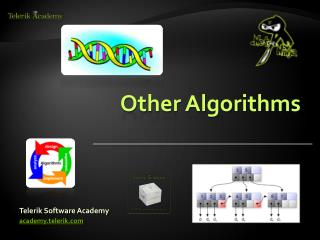DownloadDownload PresentationOther Algorithms

# Other Algorithms

Télécharger la présentation## Other Algorithms

- - - - - - - - - - - - - - - - - - - - - - - - - - - E N D - - - - - - - - - - - - - - - - - - - - - - - - - - -
##### Presentation Transcript

3. Heuristics

4. Heuristics • Solving problem more quickly than classic methods • Finding approximate solution • When optimal solution is complex and hard to find (requires time and memory) • Heuristics give simple solution (not optimal!) • Quickly produce solution that is good enoughvs finding optimal solution very very slow (mostly NP-complete problems)

5. Heuristics • Usage • anti-virus scanners • A* - Dijkstra + some cool stuff • Computes path quickly but the path might not be the shortest

6. Greedy

7. Greedy • Locally optimal choice at each stage • Finds local extremum (not always global) • Much faster than always finding optimal solution • Example: • Knapsack • Travelling salesman • Prim and Kruskal • Etc.

8. Greedy • What do we need: • Candidate set • Selection function • Feasibility function • Objective function • Solution function • The result is NOT the optimal solution

9. Greedy Live Demo

10. Genetic Algorithms

11. Genetic Algorithm • Part of Artificial Intelligence • Mimics the process of natural evolution • Terminology • Population • Fitness • Operators • Crossover • Mutation

12. Genetic Algorithm • Crossover • Mutation

13. Genetic Algorithm - Steps • Create population • Do many times (at least for 100 generations) • Determine fitness of each individual • Select next generation • Crossover • Mutation • Back to 1 • Display results

14. Genetic Algorithm Live Demo

15. Randomization

16. Randomization • Process of making something random • Monte Carlo and Las Vegas algorithms • Examples: • Generate random permutation of a sequence • Select a random sample of population • Generate random numbers

17. Geometry

18. Geometry • Vectors • Sum • Subtract • Normalization • Dot product • Cross product

19. Geometry • Distance between two points • Line equation • Intersection of two lines • Used very much in computer games and raytracing